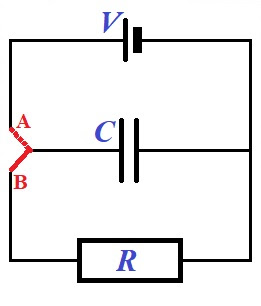## Tuesday, September 29, 2020

### UNIZOR.COM - Physics4Teens - Electromagnetism - Ohm's Law - Problems 4

Notes to a video lecture on http://www.unizor.com

Direct Current - Ohm's Law - Problems 4

Problem A

Given a circuit presented on a picture below.Initially, a red switch is in position A to fully charge a capacitor of capacity C from a battery producing a direct current with voltage V.

When a capacitor is fully charged, a switch is moved to position B, disconnecting a capacitor from a battery and forming a new circuit that includes only a fully charged capacitor and a resistor of resistance R.

When a switch is in position B, a
capacitor starts discharging its charge through a resistor. It's charge
will gradually diminish to zero, when all excess electrons on its one
plate will flow through a resistor to a plate with deficiency of
electrons.

During this process of discharge the electric current in a circuit that
contains a capacitor and a resistor will change from some maximum value
in the beginning of this process to zero, when the discharge is
completed.

Find the charge on a capacitor Q(t) and an electric current flowing trough a resistor I(t) as functions of time t.

Solution

Assume, our switch is in position A, and we are at the charging stage, when the battery of voltage V is charging a capacitor of capacity C.

The capacity of a capacitor is defined as the constant ratio of a charge
accumulated by a capacitor to a voltage applied to its plate (see
"Capacitors" lecture in the "Electromagnetism - Electric Field"
chapter):

C = Q/V

Therefore, the full charge of a capacitor at the end of the first stage of charging is V·C.

Then we flip a switch into position B, starting the second stage - discharging of a capacitor through a resistor.

At the beginning of this second stage a capacitor is fully charged. So, at time t=0 its charge is

Q(0) = V·C

The charge on a capacitor at any time produces a voltage between its plates

V(t) = Q(t)/C

This voltage produces a current flowing through a resistor I(t) that, according to the Ohm's Law, should be equal to

I(t) = V(t)/R

From the two equations above we conclude

Q(t)/C = I(t)·R

This is our first equation that connects two time-dependent (that is,
functions of time) variables - an electric current in a circuit I(t) and a charge on a capacitor Q(t).

The second functional equation is, basically, a definition of an
electric current as the rate of electric charge flowing in a circuit
(that is, amperage is how much electricity in coulombs flows through a circuit per unit of time - a second).

Mathematically speaking, an electric current is the first derivative of
an electric charge by time, taken with a sign that depends on the
direction of the change of the charge (plus if the charge is increasing and minus if decreasing):

I(t) = −dQ(t)/dt

Considering the charge Q(t) is decreasing and, therefore,
its derivative is negative, while we would like the electric current to a
be a positive number, we have to use a minus sign in this equation.

This is our second functional equation (that happens to be differential)
connecting two functions - an electric current in a circuit I(t) and a charge on a capacitor Q(t).

Now we have two functional equations, one of them is differential, and an initial condition:

Q(t)/C = I(t)·R

I(t) = −dQ(t)/dt

Q(0) = V·C

It's up to our mathematical skills to solve this system of equations.

First, we substitute I(t) from the second equation into the first, getting a differential equation for Q(t)

Q(t)/C = −R·dQ(t)/dt

This can be converted into

dQ(t)/Q(t) = −dt/(R·C)

or

d[ln(Q(t))] = d[−t/(R·C)]

If differentials of two functions are equal, the functions themselves
are just separated by a constant that can be determined using the
initial condition. Let denote that constant as K.

ln(Q(t)) = −t/(R·C) + K

or, applying an exponent to both sides of this equation,

Q(t) = eK·e−t/(R·C)

It's time to use the initial condition Q(0)=V·C to determine the multiplier eK.

For t=0 the right side of an expression for Q(t) equals to eK. Therefore, this multiplier equals to V·C.

Therefore, the final expression for a charge on a capacitor as a function of time Q(t) is

Q(t) = V·C·e−t/(R·C)

So, a charge on a capacitor is exponentially diminishing.

From the expression of Q(t) we can find the expression on an electric current going through a resistor, using the equation

I(t) = −dQ(t)/dt

from which follows

I(t) = −d[V·C·e−t/(R·C)]/dt =

= −V·C·
d
[e−t/(R·C)]/dt =

= −(V·C)·(−1/(R·C))·e−t/(R·C) =

= (V/R)·e−t/(R·C)

Q(t) = (V·C)·e−t/(R·C)

The multiplier V·C is the initial full charge of a capacitor.

I(t) = (V/R)·e−t/(R·C)

The multiplier V/R is the current that would flow through a resistor, if there were no capacitor. This follows from the Ohm's Law.